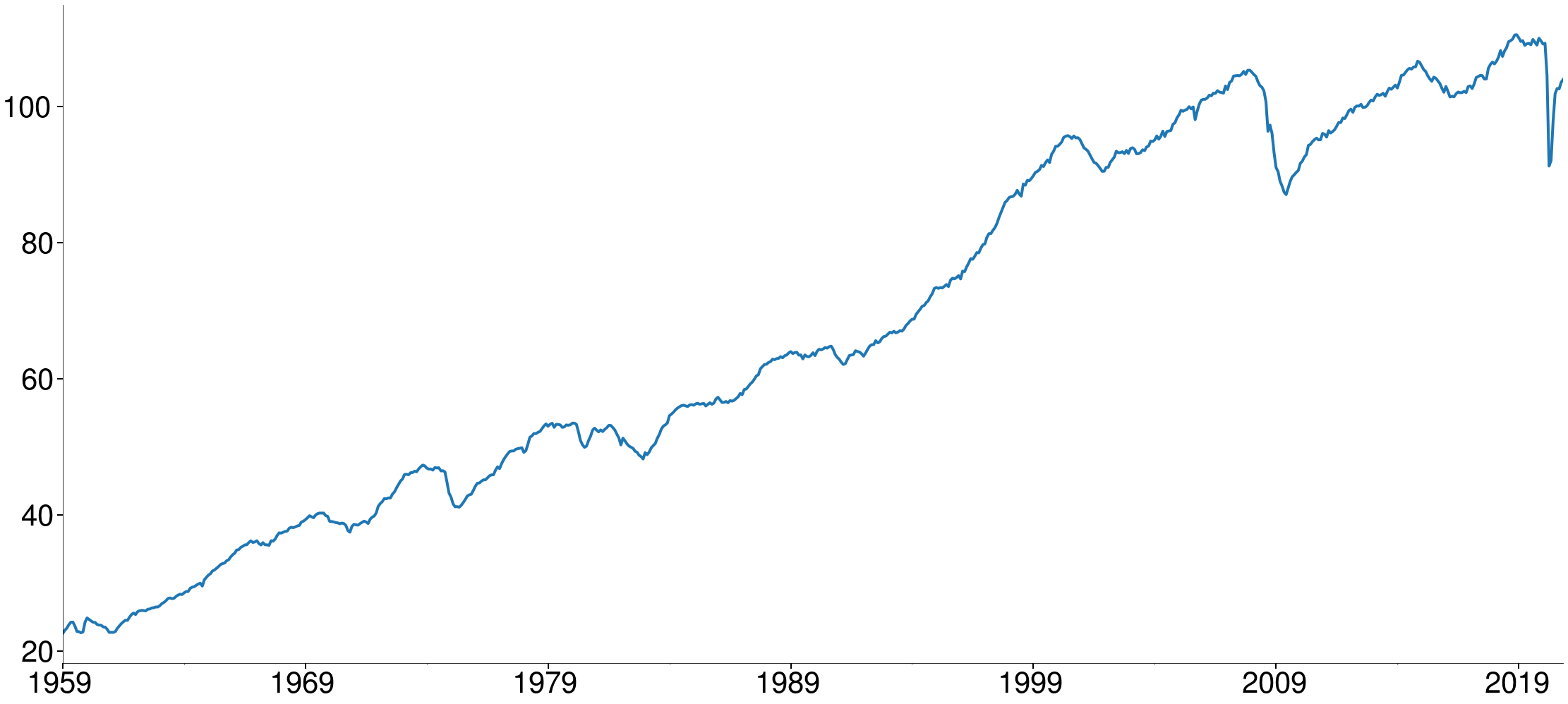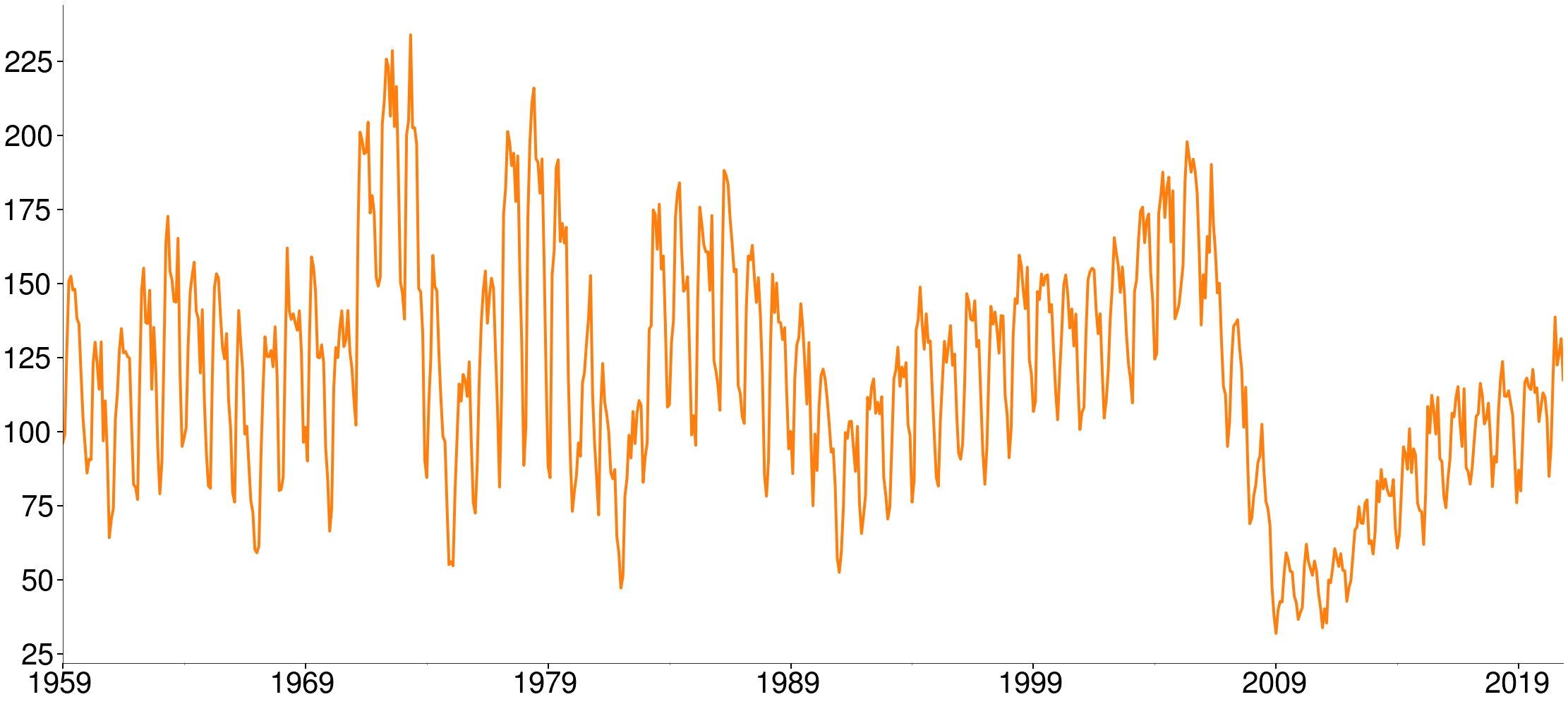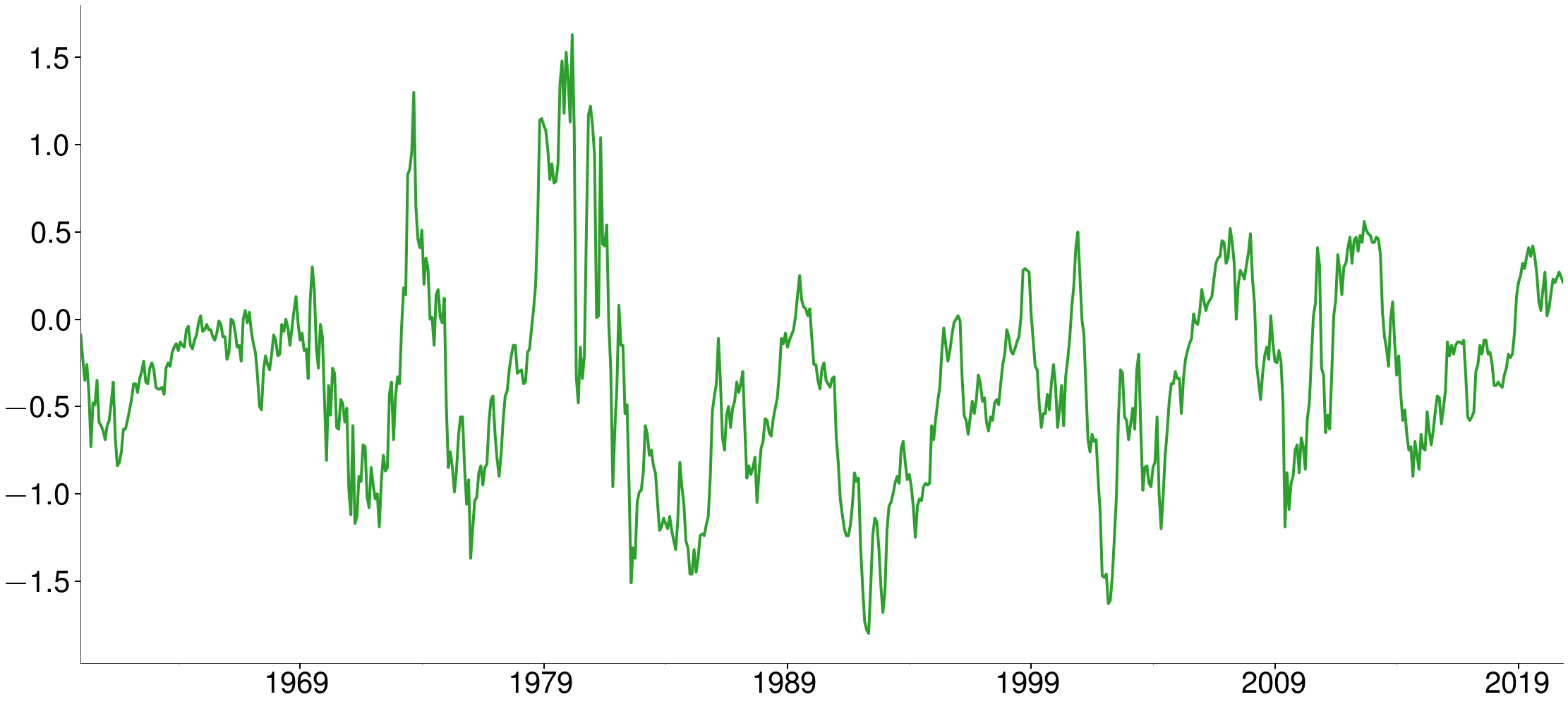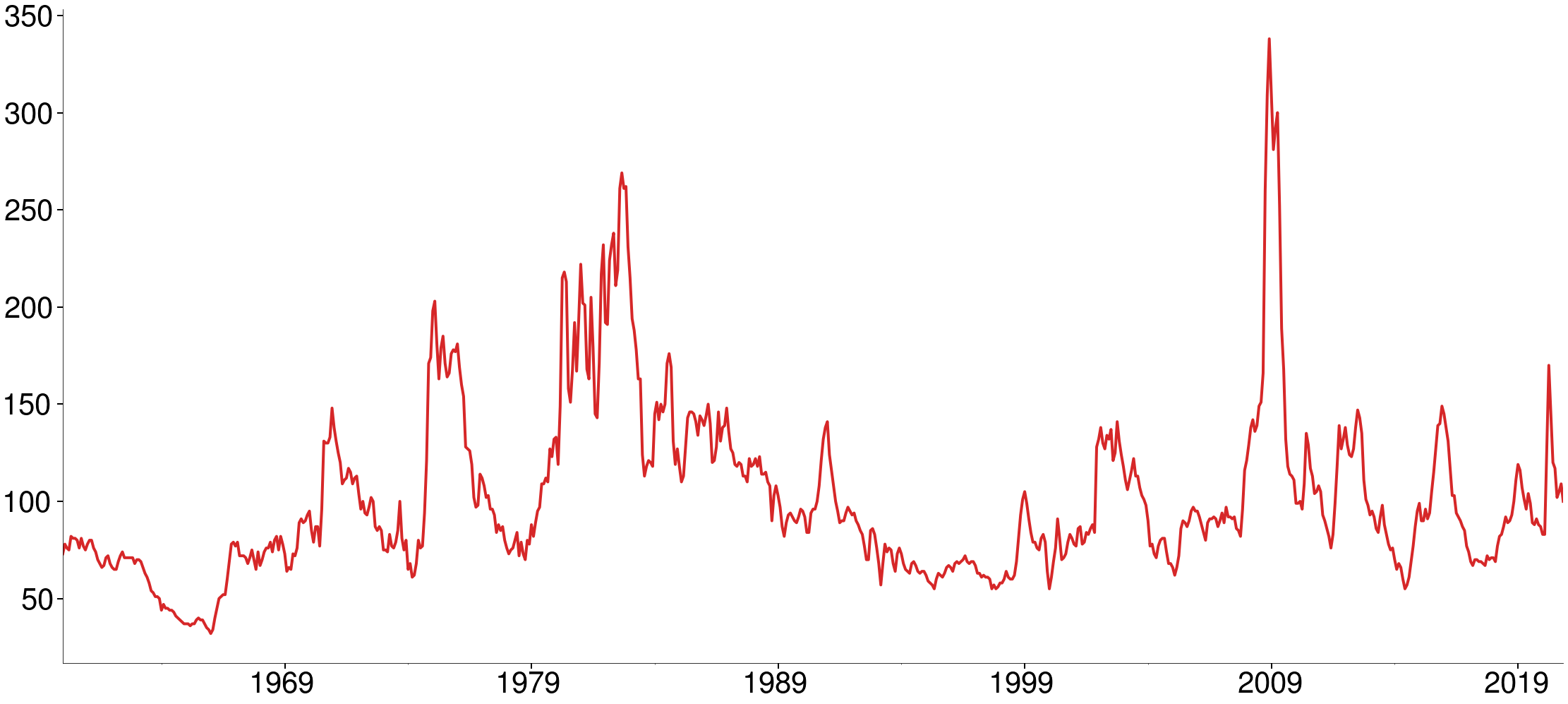# Data¶

• All data from the Federal Reserve Economics Database (FRED) excluding the VWM return.
• Data from 1959 until end of 2020.

### Series¶

• Industrial Production
• Curvature of the Yield Curve
$$\left(Y_{10}-Y_5\right)-\left(Y_{5}-Y_{1}\right)$$
• Default Rate
$$Y_{Baa} - Y_{Aaa}$$
• US Construction workers
• Housing Starts (Not Seasonally Adjusted)
• Value-Weighted Market Return (CRSP)
• Simulated AR(2)
$$Y_t = 10 + 1.4 Y_{t-1} - 0.5 Y_{t-2} + \epsilon_t$$

# Industrial Production Index¶

In :
plot(orig.INDPRO)# Housing Starts (NSA)¶

In :
plot(orig.HOUSTNSA)# Curvature of the Yield Curve¶

In :
plot(curve)plot(default)plot(orig.USCONS)